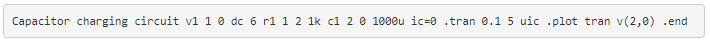# 3.17: Capacitor Charging and Discharging

In this experiment, we will aim to learn about the following concepts:

• Capacitor charging action
• Capacitor discharging action
• Time constant calculation
• Series and parallel capacitance

## Capacitor Charging and Discharging Experiment Parts and Materials

To do this experiment, you will need the following:

• 6-volt battery
• Two large electrolytic capacitors, 1000 µF minimum (Radio Shack catalog # 272-1019, 272-1032, or equivalent)
• Two 1 kΩ resistors
• One toggle switch, SPST (“Single-Pole, Single-Throw”)

Large-value capacitors are required for this experiment to produce time constants slow enough to track with a voltmeter and stopwatch. Be warned that most large capacitors are of the “electrolytic” type, and they are polarity sensitive! One terminal of each capacitor should be marked with a definite polarity sign. Usually, capacitors of the size specified to have a negative (-) marking or series of negative markings pointing toward the negative terminal. Very large capacitors are often polarity-labeled by a positive (+) marking next to one terminal.

Failure to heed proper polarity will almost surely result in capacitor failure, even with a source voltage as low as 6 volts. When electrolytic capacitors fail, they typically explode, spewing caustic chemicals and emitting foul odors. Please, try to avoid this!

I recommend a household light switch for the “SPST toggle switch” specified in the parts list.

• Lessons In Electric Circuits, Volume 1, chapter 13: “Capacitors”
• Lessons In Electric Circuits, Volume 1, chapter 16: “RC and L/R Time Constants”

## Charging and Discharging Circuit Schematic Diagram## Illustration of the Experiment## Experiment Instructions

### Measuring Voltage of Your Circuit

Build the “charging” circuit and measure voltage across the capacitor when the switch is closed. Notice how it increases slowly over time, rather than suddenly as would be the case with a resistor. You can “reset” the capacitor back to a voltage of zero by shorting across its terminals with a piece of wire.

The “time constant” (τ) of a resistor-capacitor circuit is calculated by taking the circuit resistance and multiplying it by the circuit capacitance. For a 1 kΩ resistor and a 1000 µF capacitor, the time constant should be 1 second. This is the amount of time it takes for the capacitor voltage to increase approximately 63.2% from its present value to its final value: the voltage of the battery.

It is educational to plot the voltage of a charging capacitor over time on a sheet of graph paper, to see how the inverse exponential curve develops. In order to plot the action of this circuit, though, we must find a way of slowing it down. A one-second time constant doesn’t provide much time to take voltmeter readings!

### Changing a Circuit’s Time Constant

We can increase this circuit’s time constant two different ways:

• Changing the total circuit resistance, and/or
• Changing the total circuit capacitance.

Given a pair of identical resistors and a pair of identical capacitors, experiment with various series and parallel combinations to obtain the slowest charging action. You should already know by now how multiple resistors need to be connected to form a greater total resistance, but what about capacitors?

This circuit will demonstrate to you how capacitance changes with series and parallel capacitor connections. Just be sure that you insert the capacitor(s) in the proper direction: with the ends labeled negative (-) electrically “closest” to the battery’s negative terminal!

The discharging circuit provides the same kind of changing capacitor voltage, except this time the voltage jumps to full battery voltage when the switch closes and slowly falls when the switch is opened.

Experiment once again with different combinations of resistors and capacitors, making sure as always that the capacitor’s polarity is correct.

## Computer Simulation

### Schematic with SPICE node numbers:Netlist (make a text file containing the following text, verbatim):